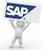# ABAP – Get max value of variable type as PACKED numberIn this short code snippet I try to get maximum number of a variable typed as packed number and compare it against given amount.

This code can be used to detect possible overflow and let program react accordingly.

```CLASS lcl_amount_checks DEFINITION.
PUBLIC SECTION.
CLASS-METHODS:
check_max_amount
IMPORTING  iv_pack_length   TYPE i DEFAULT 7
iv_pack_decimals TYPE i DEFAULT 2
iv_amount        TYPE p
EXCEPTIONS amount_too_big.
ENDCLASS.

CLASS lcl_amount_checks IMPLEMENTATION.
METHOD check_max_amount.
DATA:
lr_amount TYPE REF TO data.

CREATE DATA lr_amount
TYPE p LENGTH iv_pack_length
DECIMALS iv_pack_decimals.
ASSIGN lr_amount->* TO FIELD-SYMBOL(<lv_amount>).

*   GET MAX amount for P(LENGTH,DECIMALS)
DATA(lr_max_value) = cl_abap_exceptional_values=>get_max_value(
<lv_amount> ).
ASSIGN lr_max_value->* TO <lv_amount>.

IF iv_amount GT <lv_amount>.
MESSAGE 'Amount too large' type 'E' RAISING amount_too_big.
ENDIF.
ENDMETHOD.
ENDCLASS.```